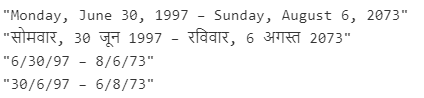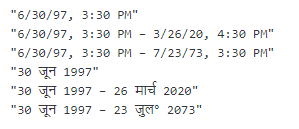Related Articles

# JavaScript | Intl.DateTimeFormat.prototype.formatRange() Method

• Last Updated : 31 Mar, 2020

The Intl.DateTimeFormat.prototype.formatRange() method is an inbuilt method in JavaScript which is used to formats a date range in the most concise way based on the locale and options provided when instantiating Intl.DateTimeFormat object.

Syntax:

Hey geek! The constant emerging technologies in the world of web development always keeps the excitement for this subject through the roof. But before you tackle the big projects, we suggest you start by learning the basics. Kickstart your web development journey by learning JS concepts with our JavaScript Course. Now at it's lowest price ever!

`Intl.DateTimeFormat.prototype.formatRange(startDate, endDate)`

Parameters: This method accept two parameters as mentioned above and described below:

• startDate: This parameter holds the starting date of the range.
• endDate: This parameter holds the ending date of the range.

Below examples illustrate the Intl.DateTimeFormat.prototype.formatRange() method in JavaScript:

Example 1:

 `const geeks1 = { weekday: ``'long'``, year: ``'numeric'``, ``                 ``month: ``'long'``, day: ``'numeric'` `};``const geeks2 = { year: ``'2-digit'``, month: ``'numeric'``, ``                 ``day: ``'numeric'` `};`` ` `const startDate = ``new` `Date(Date.UTC(1997, 5, 30, 3, 3, 3));``const endDate = ``new` `Date(Date.UTC(2073, 7, 6, 11, 0, 0));`` ` `const dateTime = ``new` `Intl.DateTimeFormat(``'en'``, geeks1);``console.log(dateTime.formatRange(startDate, endDate));`` ` `const dateTime1 = ``new` `Intl.DateTimeFormat(``'hi'``, geeks1);``console.log(dateTime1.formatRange(startDate, endDate));`` ` `const dateTime2 = ``new` `Intl.DateTimeFormat(``'en'``, geeks2);``console.log(dateTime2.formatRange(startDate, endDate));`` ` `const dateTime3 = ``new` `Intl.DateTimeFormat(``'hi'``, geeks2);``console.log(dateTime3.formatRange(startDate, endDate));`

Output:Example 2:

 `let geek1 = ``new` `Date(Date.UTC(1997, 5, 30, 10, 0, 0));``let geek2 = ``new` `Date(Date.UTC(2020, 2, 26, 11, 0, 0));``let geek3 = ``new` `Date(Date.UTC(2073, 6, 23, 10, 0, 0));`` ` `let result1 = ``new` `Intl.DateTimeFormat(``"en"``, {``    ``year: ``'2-digit'``,``    ``month: ``'numeric'``,``    ``day: ``'numeric'``,``    ``hour: ``'numeric'``,``    ``minute: ``'numeric'``});``console.log(result1.format(geek1));``console.log(result1.formatRange(geek1, geek2));``console.log(result1.formatRange(geek1, geek3));`` ` `let result2 = ``new` `Intl.DateTimeFormat(``"hi"``, {``    ``year: ``'numeric'``,``    ``month: ``'short'``,``    ``day: ``'numeric'``});``console.log(result2.format(geek1));``console.log(result2.formatRange(geek1, geek2));``console.log(result2.formatRange(geek1, geek3));`

Output:Supported Browsers: The browsers supported by Intl.DateTimeFormat.prototype.formatRange() method are listed below: